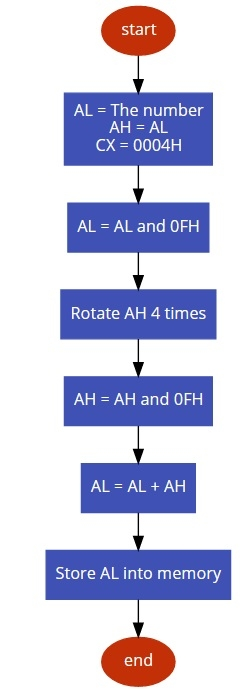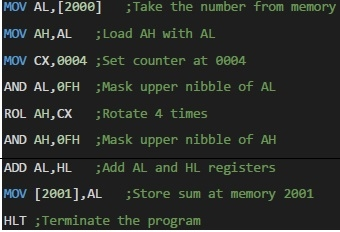# 8086 program to find sum of digits of 8 bit number

In this program we will see how to add the digits of an 8-bit number.

## Problem Statement

Write 8086 Assembly language program to add the digits of an 8-bit number stored in memory address 2000H.

## Discussion

To get the digits of an 8-bit number, we can use the masking operation. At first we will mask the upper nibble, and then the lower nibble. After masking the upper nibble, we have to rotate it to the right to make it least significant nibble. Then we can simply add it to the stored nibble to get the sum.

Data

2000
8A

## Flow Diagram## ProgramData

2001
12